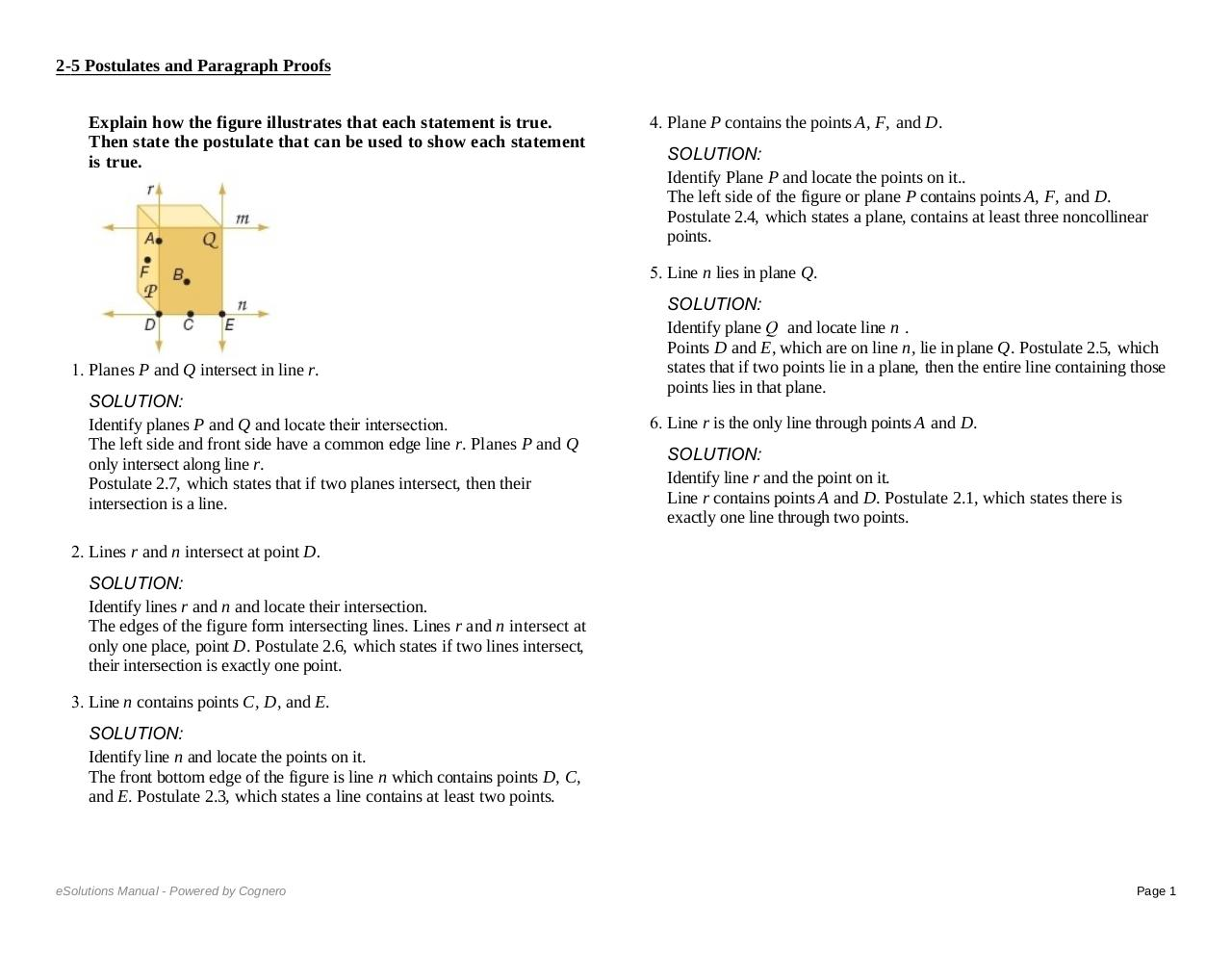# 2 5ALL.pdfPage 12318

#### Text preview

2-5 Postulates and Paragraph Proofs
Explain how the figure illustrates that each statement is true.
Then state the postulate that can be used to show each statement
is true.

SOLUTION:
Identify line n and locate the points on it.
The front bottom edge of the figure is line n which contains points D, C,
and E. Postulate 2.3, which states a line contains at least two points.
4. Plane P contains the points A, F, and D.
SOLUTION:
Identify Plane P and locate the points on it..
The left side of the figure or plane P contains points A, F, and D.
Postulate 2.4, which states a plane, contains at least three noncollinear
points.
5. Line n lies in plane Q.

1. Planes P and Q intersect in line r.
SOLUTION:
Identify planes P and Q and locate their intersection.
The left side and front side have a common edge line r. Planes P and Q
only intersect along line r.
Postulate 2.7, which states that if two planes intersect, then their
intersection is a line.
2. Lines r and n intersect at point D.
SOLUTION:
Identify lines r and n and locate their intersection.
The edges of the figure form intersecting lines. Lines r and n intersect at
only one place, point D. Postulate 2.6, which states if two lines intersect,
their intersection is exactly one point.
3. Line n contains points C, D, and E.

SOLUTION:
Identify plane Q  and locate line n .
Points D and E, which are on line n, lie in plane Q. Postulate 2.5, which
states that if two points lie in a plane, then the entire line containing those
points lies in that plane.
6. Line r is the only line through points A and D.
SOLUTION:
Identify line r and the point on it.
Line r contains points A and D. Postulate 2.1, which states there is
exactly one line through two points.
Determine whether each statement is always, sometimes, or never
7. The intersection of three planes is a line.
SOLUTION:
If three planes intersect, then their intersection may be a line or a point.
Postulate 2.7 states that two planes intersect, then their intersection is a
line. Therefore, the statement is sometimes true.

SOLUTION:
Identify line n and locate the points on it.
The front bottom edge of the figure is line n which contains points D, C,
and E. Postulate 2.3, which states a line contains at least two points.
4. Plane P contains the points A, F, and D.
SOLUTION:
Identify
Plane
P andbylocate
the points on it..
eSolutions
Manual
- Powered
Cognero
The left side of the figure or plane P contains points A, F, and D.
Postulate 2.4, which states a plane, contains at least three noncollinear

Page 1